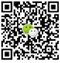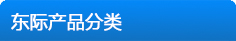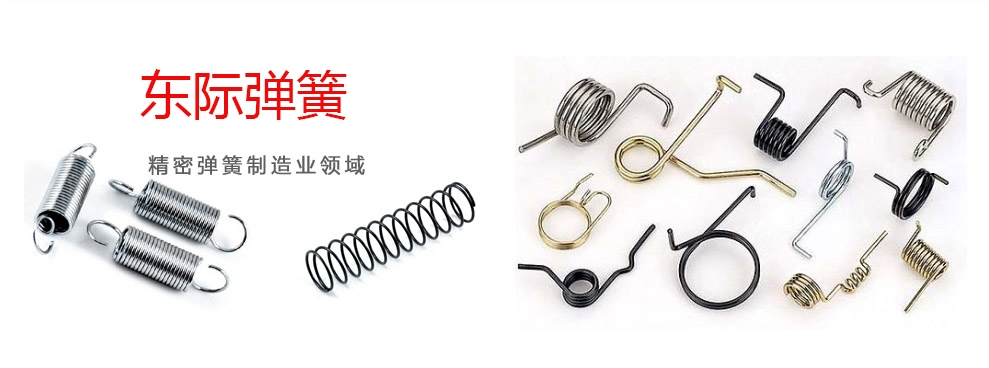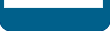扫码加微信
﻿# 怎么去设计计算一个合理的压簧？设计百科小知识都在这里啦！c = 压簧直径比 (c=D/d; c=D/b) [-]
b = 线宽 [mm, in]
d = 线径 [mm, in]
D = 中心直径 [mm, in]
F = 压簧负载 [N, lb]
G = 剪切弹性模量[MPa, psi]
h = 线高 [mm, in]
k = 压簧系数 [N/mm, lb/in]
Ks= 曲线校正因数 [-]
L0 = 自由长度 [mm, in]
LS = 固体高度 [mm, in]
n = 有效圈数 [-]
p = 节距[mm, in]
s = 压簧变形[mm, in]
Ø =形状系数 t [-] (e.g. DIN 2090)
t =压簧材料扭应力 [MPa, psi]﻿刘经理李小姐卢小姐扫二维码进手机官网